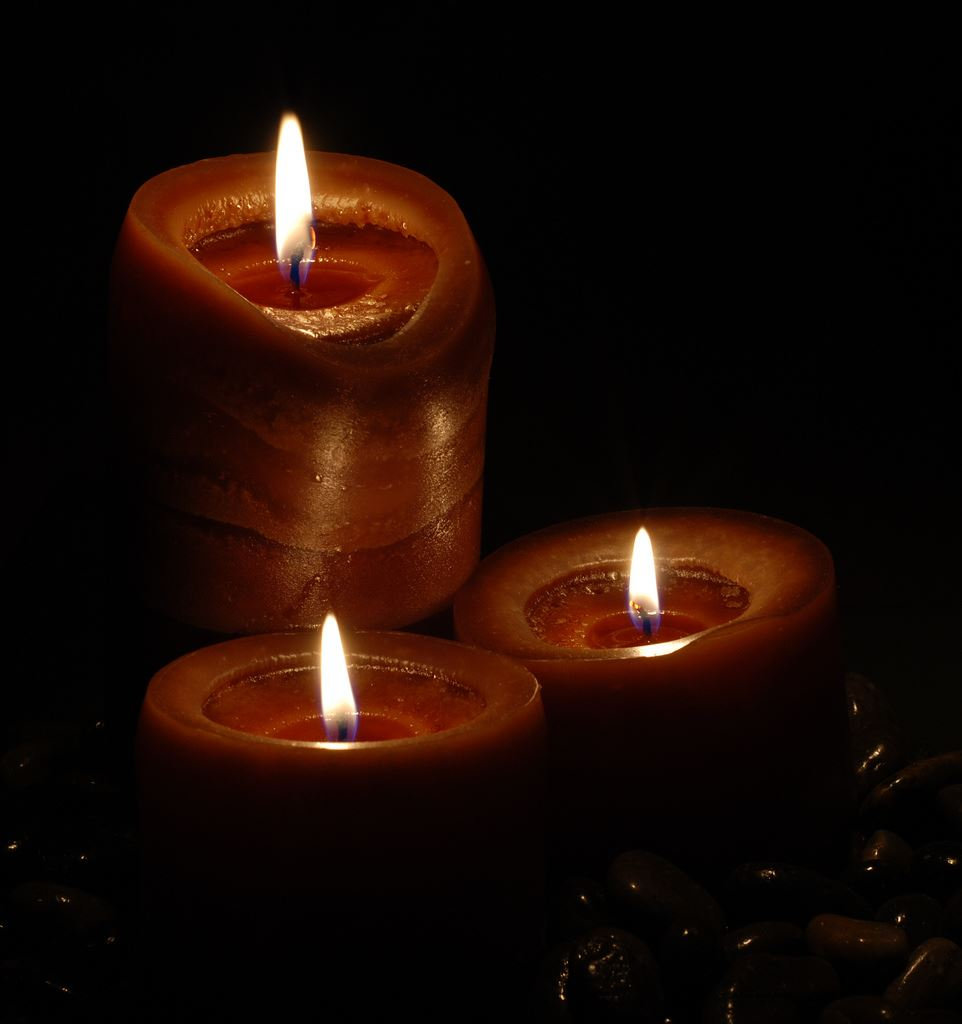# Conversion Of Solid From One Shape To Another

You might have come across several instances in your day to day life when an object is required to be converted into a different shape or size. Say your mom has a gold bar. She wants to get a ring cast out of it. What does she do? She takes it to a gold merchant to melt the gold bar and mould it into a ring. Similarly, you see wax candles of different shapes. If you want to convert a cylindrical candle into a cube, you melt the wax and pour it into a cube-shaped mould. Likewise, the conversion of solids from one shape to another is required for various purposes in daily life.#### Conversion of Solid from One Shape to Another

Each and every solid that exists occupies some volume. When you convert one solid shape to another, its volume remains the same, no matter how different the new shape is. In fact, if you melt one big cylindrical candle to 5 small cylindrical candles, the sum of the volumes of the smaller candles is equal to the volume of the bigger candle.

Hence, when you convert one solid shape to another, all you need to remember is that the volume of the original as well as the new solid remains the same. Let us discuss some examples to understand this better.

#### Examples

1. An iron ball of radius 21 cm is melted and recast into 27 spherical balls of the same radius. Find the radius of the each spherical ball.

Solution:

Volume of the iron ball = 4/3 πr3 = 4/3 x 22/7 x 21 x 21 x 21 = 38,808 cm3

Let us assume that the radius of the smaller balls is r.

So volume of 27 smaller balls = 27 x 4/3 πr3 = 36πr3

Thus we have, volume of the big iron ball = volume of 27 smaller balls

Or 38,808 cm3 = 36πr3

Or r3 = 343 cm3

So r = 7 cm

1. How many cylindrical candles, 1.75 cm in diameter and of thickness 2 mm, must be melted to form a cuboid candle of dimensions 5.5 cm × 10 cm × 3.5 cm?

Solution:

Radius of cylindrical candle = 1.75/2 = 0.875 cm

Volume of one cylindrical candle = πr2h = π x (0.875)2 x (0.02) cm3

= π x 0.0153125 = 0.048125 cm3

Volume of cuboid candle = 5.5 x 10 x 3.5 = 192.5 cm3

Thus, number of cylindrical candles = Volume of cuboid candle/Volume of one cylindrical candle

= 192.5/0.048125 = 4000